# 【杰哥带你玩转Android自动化】学亿点有备无患的"姿势"## 0x1、引言• 了解下OCR文字识别的相关概念，使用 pytesseract库chineseocr_lite 库识别图片文字；
• 了解消息推送相关，使用 Server酱 推送消息到微信；
• 学习自动化中Python常用的 图片处理操作
• 利用Android系统中的 system/bin/uiautomator.jar包，导出当前页面的所有控件信息，并对xml内容进行解析；## 0x2、OCR文字识别

OCR，Optical Character Recognition，译作 光学字符识别，亦称 计算机文字识别，指的是利用光学技术和计算机技术，对文本资料的图像文件进行分析识别处理，获取文字及版面信息的过程。

OCR技术是实现文字快速录入的一项关键技术，应用场景可就多了：

• 车牌路牌识别；
• 证件卡片识别；
• 验证码识别；
• 古籍识别；
• PDF转Word等...• 灰度化 → 彩色图像中每个像素的颜色由 RGB三个分量 决定，取值范围0-255，对于计算机来说，这样一个像素点会有256256256=16777216种颜色的变化范围。而灰度图是一种 RGB分量相同 的特殊色图像，一个像素点的变化范围只有0-255这256种。图像灰度化的目的是为了简化矩阵，提高运算速度。
• 二值化 → 将文字与背景进一步分开，将灰度值图像信号转换为只有黑(1)和白(0)的二值图像信号，可简单理解为 "黑白化"，常用方法有：分量法、最大值法、平均值法、加权平均法等；
• 降噪 → 根据噪点的特征进行去噪；
• 倾斜校正 → 倾斜的文档图像对后期的字符分割、识别和图像压缩等工作会产生较大影响，为了保证后续处理的正确性，需要对文本图像进行倾斜检测和校正；
• 大小规范化 → 将输入的任意尺寸的文字都处理成统一尺寸的标准文字，以便与预先存储在字典中的参考模板相匹配；
• 图像平滑 → 去掉笔划上的孤立白点和笔划外部的孤立黑点，以及笔划边缘的凹凸点，使得笔划边缘变得平滑；

• 版面分析 → 将文本图像分割为不同部分，并标定各部分属性，如：文本、图像、表格；
• 版面理解 → 获取文章逻辑结构，包括各区域的逻辑属性、文章的层次关系和阅读顺序；
• 图像切分 → 对文本图像进行切分，方便对单个文字进行识别处理，有两个类型：行(列)切分和字切分；
• 特征提取 → 从单个字符图像上提取统计特征或结构特征，匹配特征库中与待识别文字相似度最高的文字；
• 模型训练 → 从大量标记预料中自动学习出图像的特征；

• 版面恢复 → 根据版面分析和OCR的结果，重构出包含文字信息和版面信息的电子文档；
• 识别校正 → 特定的语言上下文的关系，对识别结果进行校正；### ① pytesseract (不推荐)``````# 安装 pytesseract
pip install pytesseract

# 安装Tesseract (区分系统)

# Windows系统 → 可到：https://github.com/UB-Mannheim/tesseract/wiki 下载exe安装包
# 注：记得选中文包，默认只支持英文识别
# 安装完，来到tesseract.exe所在的目录，复制路径，在PATH环境变量中新增此路径。

# macOS系统可用brew安装 → brew install tesseract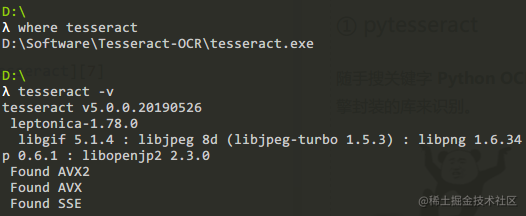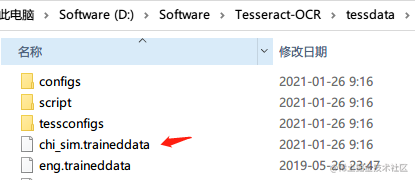``````import pytesseract
from PIL import Image

if __name__ == '__main__':
image = Image.open("test_ocr.jpg")
text = pytesseract.image_to_string(image, lang='chi_sim')
print(text)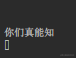em...识别率好像不是很高？尝试把文字部分抠出来 (手动截图)，再识别一下：### ② chineseocr_lite (推荐)``````from aip import AipOcr

# 读取文件内容
def get_file_content(file_path):
with open(file_path, 'rb') as fp:

class BaiDuOCR:
# 初始化，相关字段到官网控制台自行获取
def __init__(self):
self.APP_ID = "xxx"
self.API_KEY = "xxx"
self.SECRET_KEY = "xxx"
self.client = AipOcr(self.APP_ID, self.API_KEY, self.SECRET_KEY)

# 识别图片
def general(self, pic_path):
orc_result = self.client.basicGeneral(get_file_content(pic_path))
if orc_result is not None:
print("识别结果：" + str(orc_result))
else:
print("识别失败")
raise Exception("识别失败异常")

if __name__ == '__main__':
ocr_client = BaiDuOCR()
ocr_client.general('test_ocr.jpg')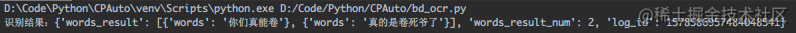``````git clone https://github.com/DayBreak-u/chineseocr_lite.git

``````python backend/main.py

``````# Python 高性能Web框架

# opencv → cv2
pip install opencv-python

# ONNX格式的机器学习模型的高性能推理引擎
pip install onnxruntime

# 小型动态图形计算库，将输入的图形路径进行处理
pip install pyclipper

# 空间几何对象库，支持点线面等集合对象及相关空间操作
pip install shapely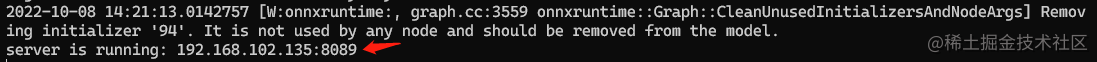``````import socket
import requests as r
from collections import OrderedDict

local_ocr_base_url = "http://{}:8089".format(socket.gethostbyname(socket.gethostname()))
local_ocr_tr_run_url = local_ocr_base_url + "/api/tr-run/"

def picture_local_ocr(pic_path):
upload_files = {'file': open(pic_path, 'rb'), 'compress': 960}
# 发送请求会自动加上Content-Type，不要手多加上，加了会报错
return extract_text(resp.json())

def extract_text(origin_data_dict):
text_dict = OrderedDict()
raw_out = origin_data_dict['data']['raw_out']
if raw_out is not None:
for raw in raw_out:
text_dict[raw] = (raw, raw, raw, raw)
return text_dict
else:
print("Json数据解析异常")

if __name__ == '__main__':
print(picture_local_ocr('test_ocr.jpg'))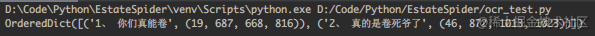## 0x3、消息推送

• 发送邮件 (免费，python中主要用到两个内置库smtplib-登录邮箱，email-构建邮件内容)
• 发送短信 (付费，调短信平台提供的API，各种传自己的信息，而且有限制)；
• 集成第三方消息推送SDK (花钱不说，可能还得自己写个接收端的APP)；
• 各种群机器人 (企业微信、钉钉、飞书、tg等)；
• 微信消息 (Server酱、微信测试号等)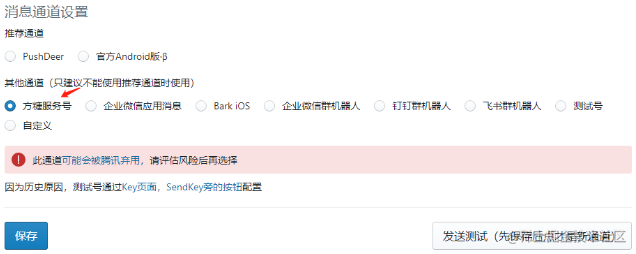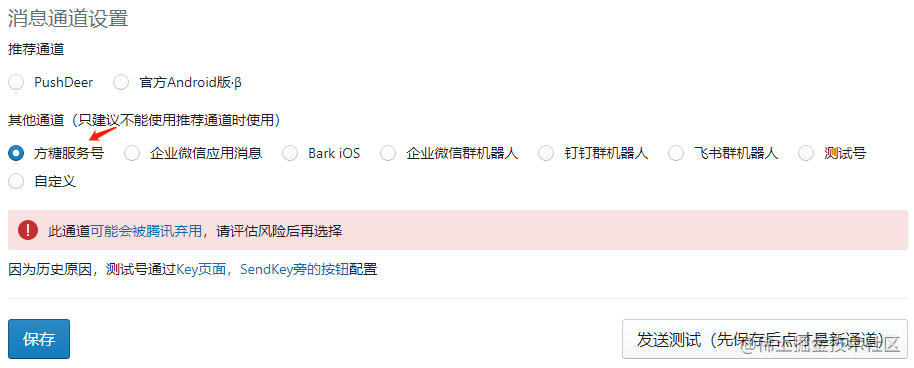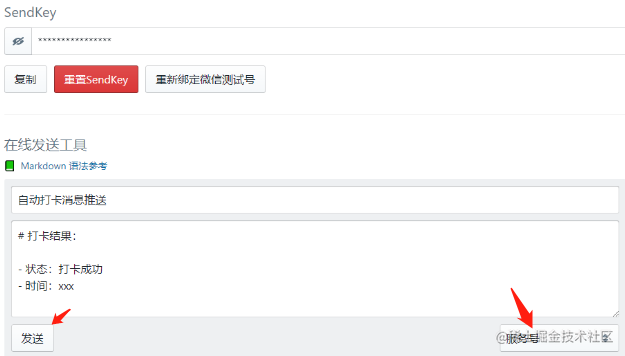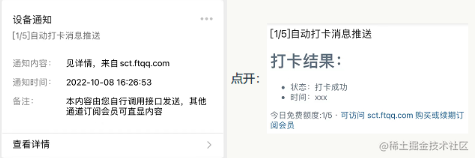``````import requests as r

send_key = "xxx"  # SendKey，官网自行获取
send_url = "https://sctapi.ftqq.com/%s.send" % send_key

def send_wx_message(title, desp, short, channel=9):
"""
发送微信消息
:param title: 标题，必填，最大长度32
:param desp: 消息内容，选填，最大长度为 32KB
:param short: 消息卡片内容，选填，最大长度64，不指定会自动截图desp的前30个显示
:param channel: 渠道，支持最多两个通道，用竖线隔开，9为方糖服务号
:return: None
"""
resp = r.post(send_url, data={'title': title, 'desp': desp, 'short': short, 'channel': channel})
if resp:
if resp.status_code == 200:
print("消息发送成功")
else:
print("消息发送失败")
print(resp.text)

if __name__ == '__main__':
send_wx_message("测试标题", "测试消息内容\n\n" * 16, "测试卡片")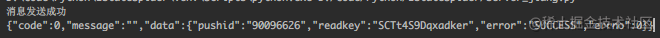## 0x4、图片处理

``````import os
import time

from PIL import Image

def get_picture_size(pic_path):
"""
获得图片尺寸
:param pic_path: 图片路径
:return: 图片宽度、图片高度，图片格式，返回样例：(1080, 1080, 'JPEG')
"""
img = Image.open(pic_path)
return img.width, img.height, img.format

def crop_area(pic_path, start_x, start_y, end_x, end_y):
"""
裁剪图片
:param pic_path: 图片路径
:param start_x: x轴起始坐标
:param start_y: y轴起始坐标
:param end_x: x轴终点坐标
:param end_y: y轴终点坐标
:return: 生成的截图路径
"""
img = Image.open(pic_path)
region = img.crop((start_x, start_y, end_x, end_y))
save_path = os.path.join(os.getcwd(), "crop_" + str(round(time.time() * 1000)) + ".jpg")
region.save(save_path)
return save_path

def resize_picture(pic_path, width, height):
"""
调整图片分辨率
:param pic_path: 图片路径
:param width: 调整后的图片宽
:param height: 调整后的图片高
:return: 调整后的图片路径
"""
img = Image.open(pic_path)
resized_img = img.resize((width, height), Image.ANTIALIAS)
save_path = os.path.join(os.getcwd(), "resized_" + str(round(time.time() * 1000)) + ".jpg")
resized_img.save(save_path)
return save_path

def resize_picture_percent(pic_path, percent):
"""
按比例调整图片分辨率
:param pic_path: 图片路径
:param percent: 缩放比例
:return: 调整后的图片路径
"""
img = Image.open(pic_path)
resized_img = img.resize((int(img.width * percent), int(img.height * percent)), Image.ANTIALIAS)
save_path = os.path.join(os.getcwd(), "resized_" + str(round(time.time() * 1000)) + ".jpg")
resized_img.save(save_path)
return save_path

def picture_to_gray(pic_path):
"""
转灰度图
:param pic_path: 图片路径
:return: 转换后的图片路径
"""
img = Image.open(pic_path)
gray_img = img.convert('L')
save_path = os.path.join(os.getcwd(), "gray_" + str(round(time.time() * 1000)) + ".jpg")
gray_img.save(save_path)
return save_path

def picture_to_black_white(pic_path):
"""
图片二值化(黑白)
:param pic_path: 图片路径
:return: 转换后的图片路径
"""
img = Image.open(pic_path)
gray_img = img.convert('1')
save_path = os.path.join(os.getcwd(), "bw_" + str(round(time.time() * 1000)) + ".jpg")
gray_img.save(save_path)
return save_path

if __name__ == '__main__':
print(get_picture_size('test_ocr.jpg'))
print(crop_area('test_ocr.jpg', 0, 0, 100, 200))
print(resize_picture('test_ocr.jpg', 300, 600))
print(resize_picture_percent('test_ocr.jpg', 0.5))
print(picture_to_gray('test_ocr.jpg'))
print(picture_to_black_white('test_ocr.jpg'))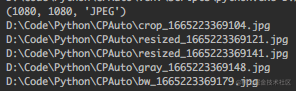## 0x5、获取当前页面所有控件信息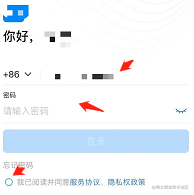• 先定位到点击账号/密码输入文本框，点击获得焦点，然后进行输入；
• 先定位到同意协议单选框，点击勾选；``````# dump出所有控件信息到xml中
adb shell /system/bin/uiautomator dump --compressed /手机存储路径/ui.xml

# 将文件从手机导出到PC

``````def current_ui_xml(save_dir=None):
"""
获取当前页面的布局xml
:param save_dir: 文件保存根目录
:return: 布局xml文件的本地路径
"""
ui_xml_name = "ui_%d.xml" % (int(round(t * 1000)))
start_cmd('adb shell /system/bin/uiautomator dump --compressed  /sdcard/%s' % ui_xml_name)
ui_xml_path = os.path.join(os.getcwd() if save_dir is None else save_dir, ui_xml_name)
start_cmd('adb pull /sdcard/%s %s' % (ui_xml_name, ui_xml_path))
return ui_xml_path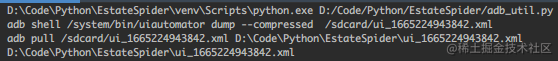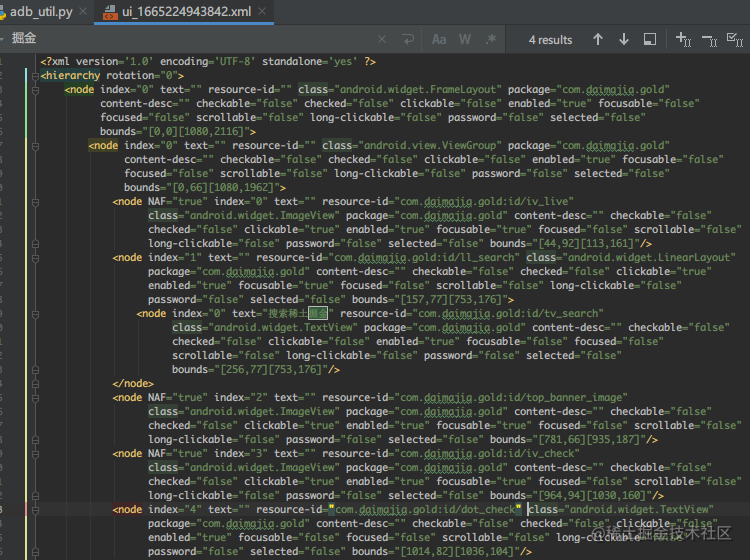``````class Node:
"""
XML节点类
"""
def __init__(self, index=None, text=None, resource_id=None, class_name=None, package=None, content_desc=None,
bounds=None):
self.index = index
self.text = text
self.resource_id = resource_id
self.class_name = class_name
self.package = package
self.content_desc = content_desc
self.bounds = bounds
self.nodes = [] # 存储子节点

self.nodes.append(node)

``````from lxml import etree
import re

bounds_pattern = re.compile(r"\[(\d+),(\d+)\]\[(\d+),(\d+)\]")  # 将坐标区域格式化为元组的正则

def analysis_ui_xml(xml_path):
"""
解析ui.xml文件
:param xml_path: xml文件路径
:return: 节点实例
"""
root = etree.parse(xml_path, parser=etree.XMLParser(encoding="utf-8"))
root_node_element = root.xpath('/hierarchy/node') # 定位到根node节点
node = analysis_element(root_node_element)
print_node(node)    # 打印看看效果
return node

def analysis_element(element):
"""
递归分析结点(转换为node对象)
:param element:
:return:
"""
if element is not None and element.tag == "node":
# 解析当前节点
bounds_result = re.search(bounds_pattern, element.attrib['bounds'])
node = Node(
int(element.attrib['index']),
element.attrib['text'],
element.attrib['resource-id'],
element.attrib['class'],
element.attrib['package'],
element.attrib['content-desc'],
(int(bounds_result), int(bounds_result), int(bounds_result), int(bounds_result))
)
# 解析子节点，递归调用
child_node_elements = element.xpath('node')
if len(child_node_elements) > 0:
for child_node_element in child_node_elements:
node_result = analysis_element(child_node_element)
if node_result:
node.nodes.append(node_result)
return node

def print_node(node, space_count=0):
"""
递归打印结点信息
:param node: 当前节点
:param space_count: 前面的空格数，区分不同层级用
:return:
"""
widget_info = "%d - %s - %s - %s - %s - %s - %s" % (
node.index, node.text, node.resource_id, node.class_name, node.package, node.content_desc, node.bounds)
print(" " * (2 * space_count), widget_info)
for child_node in node.nodes:
print_node(child_node, space_count + 1)

if __name__ == '__main__':
# 测试解析效果
analysis_ui_xml('ui_1665224943842.xml')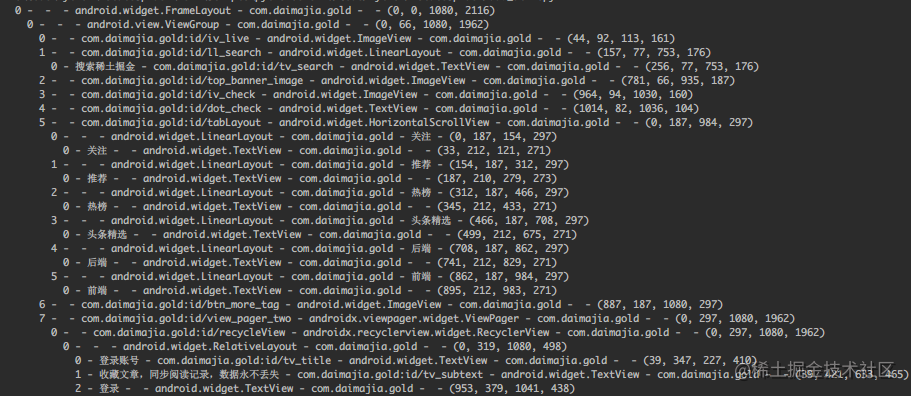## 0x6、小结Android